Balbharti Maharashtra State Board Class 10 Science Solutions Part 1 Chapter 5 Heat Notes, Textbook Exercise Important Questions and Answers.

## Maharashtra State Board Class 10 Science Solutions Part 1 Chapter 5 Heat

Question 1.
Fill in the blanks and rewrite the sentences:
a. The amount of water vapour in air is determined in terms of its………..
The amount of water vapour in air is determined in terms of its absolute humidity.

b. If objects of equal masses are given equal heat, their final temperature will be different. This is due to difference in their………….
If objects of equal masses are given equal heat, their final temperature will be different. This is due to difference in their specific heat capacities.

c. When a liquid is getting converted into solid, the latent heat is……….  (Practical Activity Sheet – 1 and 2)
When a liquid is getting converted into solid, the latent heat is released.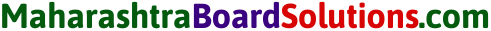Question 2.
Observe the following graph. Considering the change in volume of water as its temperature is raised from 0 °C, discuss the difference in the behaviour of water and other substances. What is this behaviour of water called?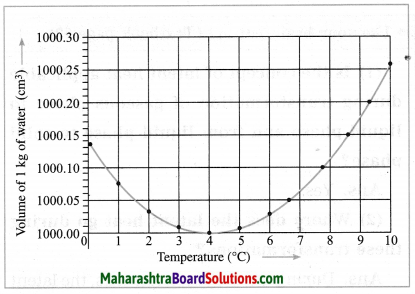If the temperature of water is raised from 0 °C to 10 °C, its volume goes on decreasing in the range 0 °C to 4 °C. It is minimum at 4 °C. The volume of water goes on increasing in the range 4 °C to 10 °C.

In general, when a substance is heated, its volume goes on increasing with temperature. Thus, in the range 0 °C to 4 °C, behaviour of water is different from other substances. It is called anomalous behaviour of water.

Question 3.
What is meant by specific heat capacity?
How will you prove experimentally that different substances have different specific heat capacities?
The amount of heat energy required to raise the temperature of a unit mass of an object by 1 °C is called the specific heat capacity of that object.

Question 4.
While deciding the unit for heat, which temperatures interval is chosen? why?
While deciding the unit for heat, the temperature interval chosen is 14.5 °C to 15.5 °C. For the reason, see the information given in the following box.Question 5.
Explain the following temperature vs time graph: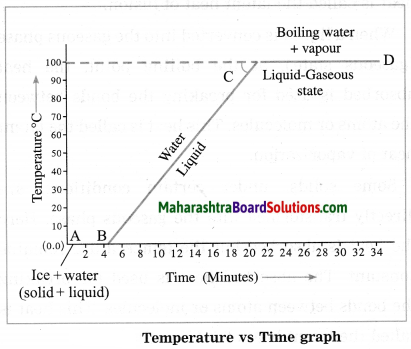(Practice Activity Sheet – 1 and 4; March 2019)
The graph shows what happens when a mixture of ice and water is heated continuously. The temperature of the mixture remains constant (0 °C) till all the ice melts as shown by the line AB. This temperature is the melting point of ice. On further heating, the temperature rises steadily from 0 °C to 100 °C as shown by the line BC, At 100 °C water starts converting into steam. This temperature is the boiling point of water. Further heating does not change the temperature and the conversion waters steam continues as shown by the line CD.

Question 6.
Explain the following:
a. the role of anomalous behaviour of water in preserving aquatic life in regions of cold climate?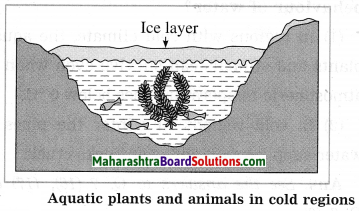In cold regions, during winter, the temperature of the atmosphere falls well below 0 °C. As the temperature decreases, the water at the surfaces of lakes and ponds starts contracting. Hence, its density increases and it sinks to the bottom. This process continues till the temperature of all the water in a lake falls to 4 °C. As the water at the surface cools further, i.e., its temperature falls below 4 °C, it starts expanding instead of contracting. Therefore, its density decreases and it remains at the surface.

The temperature of the water at the surface continues to fall to 0 °C. Finally, the water at the surface is converted into ice, but the water below the layer of ice is at 4 °C. Ice is a bad conductor of heat. Hence, the layer of the ice at the surface does not allow transfer of heat from the water to the atmosphere. As the water below the layer of ice remains at 4 °C, fish and other aquatic animals and plants can survive in it.

b. How can you relate the formation of water droplets on the outer surface of a bottle taken out of a refrigerator with formation of dew?
At a given temperature, there is a limit on how much water vapour the given volume of air can hold. The lower the temperature, the lower is the capacity of air to hold water vapour.

The temperature of a bottle kept in a refrigerator is lower than room temperature. Hence, when the bottle is taken out of the refrigerator, the temperature of the air surrounding the bottle is lowered. Therefore, the capacity of the air to hold water vapour becomes less. Hence, the excess water vapour condenses to form water droplets (like dew) on the outer surface of the bottle.

c. In cold regions in winter, the rocks crack due to anomalous expansion of water.
Sometimes water enters into crevices of the rocks. When the temperature of the atmosphere falls below 4 °C, water expands. Even when water freezes to form ice, there is increase in its volume. As there is no room for expansion, it exerts a tremendous pressure on the rocks which crack and break up into small pieces.Question 7.
a. What is meant by latent heat? How will the state of matter transform if latent heat is given off?
When a solid is heated, initially, its temperature increases. Here, the heat absorbed by the body (substance) is used in increasing the kinetic energy of the particles (atomic, molecules, etc.) of the body as well as for doing work against the forces of attraction between them. As the heating is continued, at a certain temperature (melting point), solid is converted into liquid. In this case, the temperature remains constant and the heat absorbed is used for weakening the bonds and conversion into liquid phase (liquid state). This heat is called the latent heat of fusion.

When a liquid is converted into the gaseous phase (gaseous state), at the boiling point, the heat absorbed is used for breaking the bonds between the atoms or molecules. This heat is called the latent heat of vaporization. Some solids, under certain conditions, are directly transformed into the gaseous phase. Here the heat is absorbed but the temperature remains constant. The absorbed heat is used for breaking the bonds between atoms or molecules. This heat is called the latent heat of sublimation.

In general, latent heat is the heat absorbed or given out by a substance during a change of state at constant temperature.
In transformations from liquid to solid, gas to liquid and gas to solid, latent heat is given out by the body (substance).
(Note: change of state = change of phase)

b. Which principle is used to measure the specific heat capacity of a substance?
The principle of heat exchange is used to measure the specific heat capacity of a substance. This principle is as follows: If a system of two objects is isolated from the environment by keeping it inside a heat resistant box, then no energy can leave the box or enter the box. In this situation, heat energy lost by the hot object = heat energy gained by the cold object.

c. Explain the role of latent heat in the change of state of a substance.
When a solid is heated, initially, its temperature increases. Here, the heat absorbed by the body (substance) is used in increasing the kinetic energy of the particles (atomic, molecules, etc.) of the body as well as for doing work against the forces of attraction between them. As the heating is continued, at a certain temperature (melting point), solid is converted into liquid. In this case, the temperature remains constant and the heat absorbed is used for weakening the bonds and conversion into liquid phase (liquid state). This heat is called the latent heat of fusion.

When a liquid is converted into the gaseous phase (gaseous state), at the boiling point, the heat absorbed is used for breaking the bonds between the atoms or molecules. This heat is called the latent heat of vaporization. Some solids, under certain conditions, are directly transformed into the gaseous phase. Here the heat is absorbed but the temperature remains constant. The absorbed heat is used for breaking the bonds between atoms or molecules. This heat is called the latent heat of sublimation.

In general, latent heat is the heat absorbed or given out by a substance during a change of state at constant temperature.
In transformations from liquid to solid, gas to liquid and gas to solid, latent heat is given out by the body (substance).
(Note: change of state = change of phase)

d. what basis and how will you determine whether air is saturated with vapour or not?
Whether the air is saturated with water vapour or not is determined on the basis of the extent of water vapour present in the air. If the relative humidity is 100%, the air is saturated with water vapour. In that case, we can see the formation of water droplets on the leaves of plants/grass.
If the relative humidity is less than 100%, the air is not saturated with water vapour.

Question 8.
If heat is exchanged between a hot and cold object, the temperature of the cold object goes on increasing due to gain of energy and the temperature of the hot object goes on decreasing due to loss of energy.

The change in temperature continues till the temperatures of both the objects attain the same value. In this process, the cold object gains heat energy and the hot object loses’ heat energy. If the system of both the objects is isolated from the environment by keeping it inside a heat resistant box (meaning that the energy exchange takes place between the two objects only), then no energy can flow from inside the box or come into the box.
(1) Heat is transferred from where to where?
(2) Which principle do we learn about from this process?
(3) How will you state the principle briefly?
(4) Which property of the substance is measured using this principle?
(1) Heat is transferred from a hot object to a cold object.
(2) This process shows the principle of heat exchange.
(3) In this process, the cold object gains heat energy and the hot object loses energy. If a system of two objects is isolated from the surroundings, heat energy lost by the hot object = heat energy gained by the cold object.
(4) This principle is used to measure the specific heat capacity of a substance.Question 9.
Solve the following problems:
a. Equal heat is given to two objects A and B of mass 1 g. The temperature of A increases by 3 °C and B by 5°C. Which object has more specific heat? And by what factor?
Solution:
Data: m = 1 g, Δ T1 = 3 °C, Δ T2 = 5 °C,
Q same
Here, Q = mc1 ΔT1 = mc2 ΔT2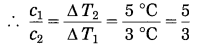Thus, c1 > c2
The specific heat of A is more than that of B and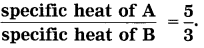b. Liquid ammonia is used in ice factory for making ice from water. If water at 20 °C is to be converted into 2 kg, ice at 0 °C, how many grams of ammonia is to be evaporated?
(Given: The latent heat of vaporization of 1 ammonia = 341 cal/g)
Solution:
Data : m1 = 2kg, ΔT1=20 °C – 0 °C
= 20 °C, c1 = 1 kcal/kg·°C, L1 (ice) = 80 kcal/kg,
L2 (vaporization of ammonia) = 341 cal/g = 341 kcal/kg, m2 =?
Q1 (heat lost by water) = m1c1 ΔT1 + m1L1
= 2kg × 1 kcal/kg·°C × 20 °C + 2 kg × 80 kcal/kg
=40 kcal + 160 kcal = 200 kcal
Q2 (heat absorbed by ammonia) = m2L2
= m2 × 34l kcal/kg
According to the principle of heat exchange, Q1 = Q2
∴ 200 kcal = m2 × 341 kcal/kg
∴ m2 = $$\frac{200}{341}$$ kg = 0.5864 kg = 586.4 g
586.4 g of ammonia are to be evaporated.

c. A thermally insulated pot has 150 g ice at temperature 0 °C. How much steam of 100 °C has to he mixed to it, so that water of temperature 50 °C will be obtained?
(Given: Latent heat of melting of ice = 80 cal/g, latent heat of vaporization of water = 540 cal/g, specific heat of water = 1 cal/g °C)
Solution:
Data: m1 = 150 g, ΔT1 = 50 °C – 0 °C
= 50 °C, cw = 1 cal/g.°C, L1 = 80 cal/g, L2 = 540 cal/g,
Δ T2 = 100°C – 50 °C = 50 °C, m2 = ?
Q1 (heat absorbed by ice) = m1L1
= 150 g × 80 cal/g = 12000 cal
Q2 (heat absorbed by water formed on melting of ice) =m1 cw ΔT1
= 150 g × 1 cal/g·°C × 50 °C = 7500 cal
Q3 (heat given out by steam) = m2L2
= m2 × 540 cal/g
Q4 (heat given out by water formed on condensation of steam)
= m2 cw ΔT2 = m2 × 1 cal/g·°C × 50 °C
According to the principle of heat exchange,
Q1 + Q2 = Q3 + Q4
∴ 12000 cal + 7500 cal = m2 × 540 cal/g + m2 × 50 cal/g
∴ 19500 cal = m2 (540 + 50) cal/g
∴ m2 = $$\frac{19500}{590}$$ g
33.5 g of steam is to be mixed.

d. A calorimeter has mass 100 g and specific heat 0.1 kcal/kg ·°C. It contains 250 g of liquid at 30 °C having specific heat of 0.4 kcal/kg·°C. If we drop a piece of ice of mass 10 g at 0 °C into the liquid, what will be the temperature of the mixture?
Solution:
Data: m1 = 100 g, c1 = 0.1 kcal/kg·°C,
= 0.1 cal/g·°C, T1 = 30 °C, m2 = 250 g,
c2 = 0.4 kcal/kg·°C = 0.4 cal/g·°C, T2 = 30 °C,
m3 = 10 g, T3 = 0 °C, L = 80 cal/g,
c (water) = 1 cal/g·°C, T = ?
Q1 (heat lost by calorimeter) = m1c1 (T- T1),
Q2 (heat lost by liquid) = m2c2 (T – T2),
Q3 (heat absorbed by ice) = m3 L,
Q4 (heat absorbed by water formed on melting of ice) = m3c (T – 0 °C)
According to the principle of heat exchange,
Q1 + Q2 = Q3 + Q4
∴ m1c1 (T1 – T) + m2c2 (T2 – T) = m3L + m3c (T – 0 °C)
∴ m1c1T1 – m1c1T + m2c2T2 – m2c2T = m3L + m3c (T – 0°C)
∴ m1c1T1 + m2c2T2 = m3L + (m1c1 + m2c2 + m3c)T
∴ 100g × 0.1 cal/g°C × 30 °C + 250g × 0.4 cal/g.°C × 30 °C J
= 10 g x× 80 cal/g + (100 g × 0.1 cal/g.°C + 250 g × 0.4 cal/g.°C + 10 g × 1 cal/g.°C) T
∴ (10 + 100 + 10) T = (300 + 3000 – 800)°C
∴ 120 T = 2500 °C
∴ T = $$\frac{2500}{120}$$ °C = $$\frac{125}{6}$$ °C = 20.83 °C
This is the temperature of the mixture.

Project:
Take help of your teachers to make a working model of Hope’s apparatus and perform the experiment. Verify the results you obtain. [Do it your self]Can you recall? (Text Book Page No. 62)

Question 1.
What is the difference between heat and temperature?
Heat is a form of energy. Particles of matter (atoms, molecules, etc.) possess potential energy and kinetic energy. Total energy (potential energy + kinetic energy) of all particles of matter in a given sample is called it’s thermal energy. When two bodies at different temperatures are in thermal contact with each other, there is transfer of thermal energy from a body at higher temperature to a body at lower temperature. This energy in transfer is called heat. It is expressed in joule, calorie and erg.

Temperature is a quantitative measure of degree of hotness or coldness of a body. It is expressed in °C, °F or K (kelvin). Temperature determines the direction of energy transfer.

Question 2.
What are the different ways of heat transfer?
Ways of heat transfer: conduction, convection and radiation.
[Note: heat ≡ heat energy. In the textbook, both the terms are used.]

Use your brain power! (Text Book Page No. 63)

Question 1.
Is the concept of latent heat applicable during transformation of gaseous phase to liquid phase and from liquid phase to solid phase?
Yes.

Question 2.
Where does the latent heat go during these transformations?
During these transformations, the latent heat is given out by the substance to the surroundings.Use your brain power! (Text Book Page No. 64)

Question 1.
In the above experiment, the wire moves through the ice slab. However, the ice slab does not break. Why?
When the thin wire with two equal weights attached to its ends is hung over the block of ice, it exerts pressure on the ice below it. Due to this, the melting point of the ice below the wire is lowered and some ice melts. The wire passes through the water so formed.

The water above the wire is no longer under pressure and, therefore, refreezes. Once again the ice below the wire melts, and the wire passes through it, and the process continues. In this way, due to alternate melting of ice and refreezing of water, the wire cuts right through the block of ice leaving the block intact.

Question 2.
Is there any relationship of latent heat with regelation?
Yes. when the ice melts, heat is absorbed, but the temperature does not change. Also, when water refreezes, heat is given out, but the temperature does not change. This heat absorbed or given out is the latent heat.

Question 3.
You know that as we go higher than the sea level, the boiling point of water decreases. What would be the effect on the melting point of a solid?
As we go higher than the sea level, the melting point of solids (i) that expand on melting is lowered due to a decrease in pressure (ii) that contract on melting is raised due to a decrease in pressure.

[The wire used in the experiment is made of a metal (usually copper). Metals are good conductors of heat. Hence, exchange of heat between the portion of the ice above the wire and that below the wire takes place readily.]

Can you tell? (Text Book Page No. 64)

Question 1.
We feel that some objects are cold, and some are hot. Is this feeling related in some way to our body temperature?
Yes. If the temperature of the object is lower than our body temperature, e.g., ice, we feel the object is cold. If the temperature of the object is higher than our body temperature, e.g., hot water, we feel the object is hot.

Use your brain power! (Text Book Page No. 66)

Question 1.
How will you explain the following statements with the help of the anomalous behaviour of water?
(1) In regions with cold climate, the aquatic plants and animals can survive even when the atmospheric temperature goes below 0 °C.
(2) In cold regions in winter the pipes for water supply break and even rocks crack.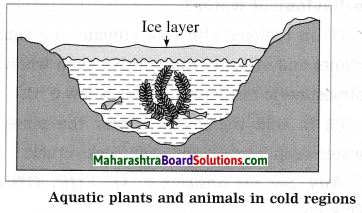In cold regions, during winter, the temperature of the atmosphere falls well below 0 °C. As the temperature decreases, the water at the surfaces of lakes and ponds starts contraeting. Hence, its density increases and it sinks to the bottom. This process continues till the temperature of all the water in a lake falls to 4 °C. As the water at the surface cools further, i.e., its temperature falls below 4 °C, it starts expanding instead of contracting. Therefore, its density decreases and it remains at the surface.

The temperature or the water at the surface continues to fall to 0 °c. Finally, the water at the surface is converted Into ice, but the water below the layer of ice is at 4 °C. Ice is a bad conductor of heat. Hence, the layer of the ice at the surface does not allow transfer of heat from the water to the atmosphere. As the water below the layer of ice remains at 4 °C, fish and other aquatic animals and plants can survive in it.(2) Sometimes water enters into crevices of the rocks. when the temperature of the atmosphere falls below 4 °C, water expands. Even when water freezes to form ice, there is increase in its volume. As there is no room for expansion, it exerts tremendous pressure on the rocks which crack and break up Into small pieces.

In cold countries, in winter, the temperature of the atmosphere falls below 0 °C. when the temperature of water falls below 4 °C, it expands. Hence, the water in pipes expands. Even if ice is rormed, there is an increase in the volume.

As there is no room for expansion, water (or ice) exerts a large pressure on the pipes. Hence, the pipelines carrying water burst.

Fill in the blanks and rewrite the sentences:

Question 1.
The amount of water vapour in air is determined in terms of its…………..
The amount of water vapour in air is determined in terms of its absolute humidity.

Question 2.
If objects of equal masses are given equal heat, their final temperature will be different. This is due to difference in their……………
If objects of equal masses are given equal heat, their final temperature will be different. This is due to difference in their specific heat capacities.

Question 3.
When a liquid is getting converted into solid, the latent heat is…………. (Practical Activity Sheet – 1 and 2)
When a liquid is getting converted into solid, the latent heat is released.

Rewrite the following statements by selecting the correct options:

Question 1.
……….is used to study the anomalous behaviour of water.
(a) Calorimeter
(b) Joule’s apparatus
(c) Hope’s apparatus
(c) Hope’s apparatus

Question 2.
When water boils and is converted into steam, then………..
(a) heat is taken in and temperature remains constant
(b) heat is taken in and temperatures rises
(c) heat is given out and temperature lowers
(d) heat is given out and temperature remains constant
(a) heat is taken in and temperature remains constant

Question 3.
When steam condenses to form water,………..
(a) heat is absorbed and temperature increases
(b) heat is absorbed and temperature remains the same
(c) heat is given out and temperature decreases
(d) heat is given out and temperature remains the same
(d) heat is given out and temperature remains the sameQuestion 4.
The temperature of ice can be decreased below 0 °C by mixing………..in it. (Practice Activity Sheet – 3)
(a) saw dust
(b) sand
(c) salt
(d) coal
(c) salt

Question 5.
Ice/water is a substance that………..
(a) expands on melting and contracts on freezing
(b) contracts on melting and does not undergo change in volume on freezing
(c) contracts on melting and expands on freezing
(d) does not undergo any change in volume on melting or freezing
(c) contracts on melting and expands on freezing

Question 6.
Heat absorbed when 1 g of ice melts at 0 °C to form 1 g of water at the same temperature is………..cal.
(a) 80
(b) 800
(c) 540
(d) 54
(a) 80

Question 7.
The latent heat of vaporization of water is………..
(a) 540 cal/g
(b) 800 cal/g
(c) 80 cal/g
(d) 54 cal/g
(a) 540 cal/g

Question 8.
The latent heat of fusion of ice is………..
(a) 540 cal/g
(b) 80 cal/g
(c) 800 cal/g
(d) 4cal/g
(b) 80 cal/g

Question 9.
If the temperature of water is decreased from 4 °C to 10 °C, then its………..
(a) volume decreases and density increases
(b) volume increases and density decreases
(c) volume decreases and density decreases
(d) volume increases and density increases
(b) volume increases and density decreases

Question 10.
At 4 °C, the density of water is………..
(a) 10 g/cm3
(b) 4g/cm3
(c) 4 × 103 kg/m3
(d) 1 × 103 kg/m3
(d) 1 × 103 kg/m3Question 11.
The density of water is maximum at………..
(a) 0 °C
(b) – 4 °C
(c) 100 °C
(d) 4 °C
(d) 4 °C

Question 12.
………..heat is needed to raise the temperature of 1 kg of water from 14.5 °C to 15.5 °C.
(a) 4180 J
(b) 103 J
(c) 1 cal
(d) 4180 cal
(a) 4180 J

Question 13.
………..heat is needed to convert 1 g of water at 0 °C and at a pressure of one atmosphere into 1 g of steam under the same conditions.
(a) 80 cal
(b) 540 cal
(c) 89 J
(d) 540 J
(b) 540 cal

Question 14.
Water expands on reducing its temperature below………..°C. (March 2019)
(a) 0
(b) 4
(c) 8
(d) 12
(b) 4

State whether the following statements are true or false. (If a statement is false, correct it and rewrite it.):

Question 1.
Specific latent heat of fusion is expressed in g/cal.
False. (Specific latent heat of fusion is expressed in cal/g.)

Question 2.
If the temperature of water is raised from 0 °C to 10 °C, its volume goes on increasing.
False. (If the temperature of water is raised from 0 °C to 10 °C, its volume goes on decreasing in the range 0 °C to 4 °C and then goes on increasing in the range 4 °C to 10 °C.)Question 3.
At dew point relative humidity is 100%.
True.

Question 4.
1 kcal = 4.18 joules.
False. (1 kcal = 4180 joules.)

Question 5.
Specific heat capacity is expressed in cal/g·°C
True.

Question 6.
Latent heat of fusion, Q = mL.
True.

Question 7.
If the relative humidity is more than 60%, we feel that the air is humid.
True.

Question 8.
If the relative humidity is less than 60%, we feel that the air is dry.
True.

Question 9.
Relative humidity has no unit.
True.Question 10.
Absolute humidity is expressed in kg/m3.
True.

Identify the odd one and give the reason:

Question 1.
Temperature. It is a physical quantity. Others are modes of transfer of heat.

Question 2.
The joule, The erg, The calorie, The newton.
The newton. It is a unit of force. Others are units of energy (as well as work.)

Question 3.
cal/g, cal/g·°C, k cal/kg·°C, erg/g·°C.
cal/g. It is a unit of specific latent heat. Others are units of specific heat capacity.

Match the columns:

 Column A Column B 1. Latent heat a. Q = mc ΔT 2. Specific heat capacity b. Q = mL 3. Heat absorbed or given out by a body when its temperature changes. c. kcal d. cal/g·°C

(1) Latent heat – Q = mL
(2) Specific heat capacity – cal/g·°C
(3) Heat absorbed or given out by a body when its temperature changes – Q = mc ΔT.

Answer the following questions in one sentence each:

Question 1.
State units of temperature.
Units of temperature: °C, °F and K (kelvin).

Question 2.
State units of energy.
Units of energy: the erg, the joule, the calorie.

Question 3.
State the relation between the joule and the calorie.
1 calorie = 4.18 joules.Question 4.
State the relation between the erg and the joule.
1 joule = 107 ergs.

Question 5.
State the relation between the erg and the kilocalorie.
1 kilocalorie = 4.18 × 1010 ergs.

Question 6.
State the relation between the joule and the kilocalorie.
1 kilocalorie = 4.18 × 103 joules.

Question 7.
When heat energy is absorbed by an object, ΔT represents the rise in temperature. What would ΔT represent if the object loses heat energy? (Practice Activity Sheet – 4)
If the object loses heat energy, ΔT would represent the decrease in temperature.

Question 1.
Define latent heat of fusion.
(OR)
What is latent heat of fusion? State its units.
When a solid is converted into liquid at constant temperature (melting point of the substance) the amount of heat absorbed by it is called the latent heat of fusion.
Heat is a form of energy. Hence, latent heat is expressed in units joule, erg, calorie or kilocalorie.

Question 2.
Define specific latent heat of fusion.
(OR)
What is specific latent heat of fusion? State its units.
The amount of heat energy absorbed at constant temperature by unit mass of a solid to convert into liquid phase is called the specific latent heat of fusion.
It is expressed in units J/kg, erg/g, cal/g, kJ/ kg and kcal/kg.

[Note: Specific latent heat (L) = $$\frac{\text { latent heat }(Q)}{\text { mass of the substance }(m)}$$
:. SI unit of specific latent heat = SI unit of energy / SI unit of mass = J/kg]Question 3.
Explain the term latent heat of vaporization.
When a liquid is heated continuously, initially, its temperature increases. Later, at a certain stage, its temperature does not increase even when heat is supplied to it. At this temperature, heat absorbed by the liquid is used for breaking the bonds between its atoms or molecules, i.e., for doing work against the forces of attraction between the atoms or molecules and conversion into gaseous phase.

This heat is called the latent heat of vaporization and the constant temperature at which this change of state occurs is called the boiling point of the liquid.

Question 4.
Define boiling point of a liquid.
(OR)
What is boiling point of a liquid?
The constant temperature at which a liquid transforms into gaseous state is called the boiling point of the liquid.
[Note: On application of pressure, the boiling point of a liquid is raised. On reducting the pressure, the boiling point is lowered.]

Question 5.
Define specific latent heat of vaporization.
OR
What is specific latent heat of vaporization?
The amount of heat energy absorbed at constant temperature by unit mass of a liquid to convert into gaseous phase is called the specific latent heat of vaporization.

Question 6.
The specific latent heat of fusion of ice is 80 cal/g. Explain this statement.
When 1 g of ice at a pressure of one atmosphere and at a temperature 0 °C is converted into 1 g of water, heat absorbed by the ice is 80 cal.Question 7.
The specific latent heat of fusion of silver is 88.2 kJ/kg. Explain this statement.
When 1 kg of silver at a pressure of one atmosphere and at a temperature of 962 °C (melting point of silver) is converted into 1 kg of silver in liquid phase, heat absorbed by the silver is 88.2 kJ.

Question 8.
The specific latent heat of vaporization of water is 540 cal/g. Explain this statement.
When 1 g of water at a pressure of one atmosphere and at a temperature of 100 °C is converted into 1 g of steam, heat absorbed by the water is 540 cal.

Question 9.
Define regelation.
(OR)
What is regelation?
The phenomenon in which the ice converts to liquid due to applied pressure and then re-converts to ice once the pressure is removed is called regelation.

Question 10.
The terms hot and cold are used in relative context. Explain.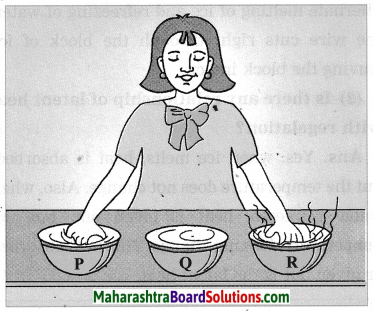(1) Take three large bowls, P, Q and R. Fill bowl P with cold water, bowl Q with lukewarm water, and bowl R with hot water.
(2) Immerse your right hand in bowl P, and left hand in bowl R for about five seconds.
(3) Now, immerse both the hands in bowl Q at the same time.
(4) You will find that the water in bowl Q appears warm to your right hand, and cold to your left hand. Thus, the hand immersed in cold water for some time finds the lukewarm water hot while the one immersed in hot water finds the same lukewarm water cold. This experiment shows that the terms hot and cold are relative.Question 11.
Draw a neat labelled diagram of Hope’s apparatus. Explain how this apparatus can be used to demonstrate anomalous behaviour of water. Draw a graph of temperature of water against time.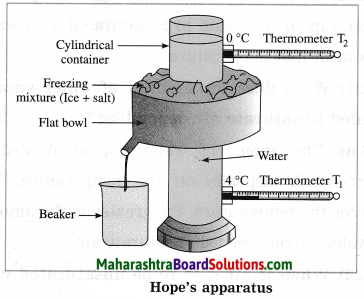The figure shows Hope’s apparatus. Initially, the cylindrical container in Hope’s apparatus is filled with water at about 12 °C and the flat bowl is filled with a freezing mixture of ice and salt.

The temperature of water in the upper part of the container (T2) is recorded by thermometer T2 and that of water in the lower part of the container (T1) is recorded by thermometer T1. Figure shows variation of temperature of water with time.

Initially, both the thermometers show the same temperature (say, 12 °C). In a short time, the temperature shown by the lower thermometer starts decreasing, while the temperature shown by the upper thermometer does not change very much.

This process continues till the temperature shown by the lower thermometer falls to 4 °C and remains constant thereafter. This shows that in the temperature range 12 °C to 4 °C, the density of the water in the central part of the container goes on increasing and hence the water sinks to the bottom. It means that water contracts, i.e., its volume decreases as its temperature falls from 12 °C to 4 °C.

As the temperature of the water in the central part of the container becomes less than 4 °C, the temperature shown by the upper thermometer begins to fall rapidly to 0 °C. But the temperature shown by the lower thermometer remains constant (4 °C). Later, the heading shown by the lower thermometer decreases to 0 °C.

In the temperature range 4 °C to 0 °C, the water moves upward. This shows that the density of water goes on decreasing in this range. It means that water expands, i.e. its volume increases as its temperature falls from 4 °C to 0 °C.

Thus, the volume of a given mass of water is minimum at 4 °C, i.e., the density of water is maximum at 4 °C.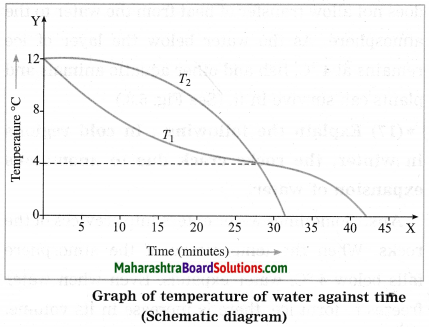In the above figure, the point of intersection of the two curves shows the temperature at which the density of water is maximum. This temperature is 4 °C.

Question 12.
A mountaineer climbing on the Everest, experienced the following facts. Explain each fact with the scientific reason : (1) He found j fishes alive below the ice (2) Time required for cooking was more as he went higher (3) He saw many times cliffs falling suddenly (4) He saw tubes carrying water broken.
Explanation:
(1) Water expands as its temperature decreases from 4 °C to 0 °C. Water is converted into ice at 0 °C. The density of water is more than that of ice. Fishes can remain alive in the water (at 4 °C) below the ice.
(2) At high altitudes, atmospheric pressure is low and hence water boils at a temperature lower than its normal boiling point. Therefore, the time required for cooking food is more at higher altitudes.
(3) Water expands while freezing. Hence, the water present in the crevices of the rocks exerts a tremendous pressure on the rocks, while freezing. Therefore, the cliffs fall.
(4) Water expands while freezing. Hence, the water in the tube exerts a large pressure on the tube, while freezing. Therefore, the tube carrying water breaks.Question 13.
What is humidity?
The moisture, i.e., the presence of water vapour, in the atmosphere is called humidity.

Question 14.
When is air said to be saturated with water vapour?
When air contains maximum possible water vapour, it is said to be saturated with water vapour at that temperature.

Question 15.
What does the amount of water vapour needed to saturate air depend on?
The extent of water vapour needed to saturate air depends on the temperature. The greater the temperature, the greater is the amount of water vapour needed to saturate air.

Question 16.
When is air said to be unsaturated with water vapour?
When air contains water vapour less than its capacity to hold water vapour at that temperature, it is said to be unsaturated with water’vapour.

Question 17.
What is dew point temperature?
(OR)
Define dew point temperature.
If the temperature of unsaturated air is decreased, a temperature is reached at which the air becomes saturated with water vapour. This temperature is called the dew point temperature.

Question 18.
Name the physical quantity used to express the amount of water vapour present in air.
Absolute humidity.Question 19.
Define absolute humidity.
(OR)
What is absolute humidity? State its unit.
The mass of water vapour present in a unit volume of air is called absolute humidity. Generally it is expressed in kg/m3.

Question 20.
Define relative humidity.
(OR)
What is relative humidity? Write the formula for % relative humidity.
The ratio of the actual mass of water vapour content in the air for a given volume and temperature to that required to make the same volume of air saturated with water vapour at the same temperature is called the relative humidity.

% Relative humidity = [the actual mass of water vapour content in the air for a given volume and temperature ÷ the mass of water vapour required to make the same volume of air saturated with water vapour at the same temperature] × 100%.

Question 21.
What is the value of relative humidity at the dew point temperature?
At the dew point temperature, relative humidity is 100%.

Question 22.
The mass of water vapour in air enclosed in a certain space is 60 g and the mass of water vapour needed to saturate the same air with water vapour under the same conditions is 100 g. What is the corresponding % relative humidity?
Here, % relative humidity = ($$\frac{60 \mathrm{g}}{100 \mathrm{g}}$$) × 100% = 60%

Question 23.
During winter, sometimes we see a white trail at the back of a flying aeroplane in a clear sky. Explain why.
In winter, air temperature is low. Hence, when an aeroplane flies, the vapour released by its engine condenses and forms white clouds. If the relative humidity of the air surrounding the plane is high, we see this white trail at the back of the plane for a long time before it disappears. If.the relative humidity is low, the white trail is short and disappears quickly. If the relative humidity is very low, there is no formation of the white trail.Question 24.
State two effects of humidity present in atmosphere.
Effects of humidity present in atmosphere: When the temperature of air falls below the dew point, dew and fog are formed.

Question 25.
Explain how dew and fog are formed.
(OR)
Write a short note on formation of dew and fog.
At a particular temperature, a given volume of air can contain a certain maximum amount of water vapour. Normally, the temperature of air during the day is such that air is not saturated with water vapour present in it.

As the temperature falls, the capacity of air to hold water vapour becomes less. During a cold night, the temperature of air may fall to the dew point, or even below the dew point. If the temperature falls below the dew point, the excess of water vapour in air condenses on the surfaces of cold bodies and dew is formed. If the water vapour condenses on the fine dust particles present in the atmosphere, mist or fog is formed.

Question 26.
State the units of heat.
Units of heat: joule, erg, calorie, kilocalorie.

Question 27.
Define the kilocalorie.
The amount of heat necessary to raise the temperature of 1 kg of water by 1 °C from 14.5 °C to 15.5 °C is called one kilocalorie.

Question 28.
Define the calorie.
The amount of heat necessary to raise the temperature of 1 g of water by 1 °C from 14.5 °C to 15.5 °C is called one calorie.

Question 29.
State the relation between the kilocalorie and the calorie.
1 kilocalorie = 103 calories.Question 30.
Study the following procedure and answer the questions below:  (Practice Activity Sheet – 2)
1. Take 3 spheres of iron, copper and lead of equal mass.
2. Put all the 3 spheres in boiling water in a beaker for some time.
3. Take 3 spheres out of the water. Put them immediately on a thick slab of wax.
4. Note the depth that each sphere goes into the wax.
(i) Which property of a substance can be studied with this procedure?
(ii) Describe that property in minimum words.
(iii) Explain the rule of heat exchange with this property.
(i) Specific heat.
(ii) Specific heat: The amount of heat energy required to raise the temperature of a unit mass of an object by 1 °C.
(iii) According to the rule/principle of heat exchange, heat energy lost by the hot object = heat energy gained by the cold object.

In this activity, heat absorbed by the iron sphere is transmitted more in the wax, hence the sphere goes deepest into the wax, while the lead sphere absorbs less heat, resulting in less transmission of heat in the wax, hence, the sphere goes the least depth into the wax.

Question 31.
Write the symbol for specific heat capacity. State the units of specific heat capacity.
Symbol for specific heat capacity: c.
Units of specific heat capacity: J/kg·°C,
erg/g·°C, cal/g·°C, kcal/kg·°C.
[ Notes: (1) Specific heat capacity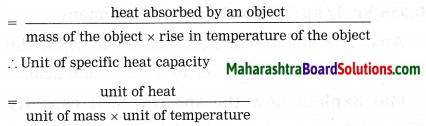In SI, heat is expressed in joule (J), mass in kg and temperature in kelvin(K).
∴ SI unit of specific heat capacity = $$\frac{\mathrm{J}}{\mathrm{kg} \cdot \mathrm{K}}$$. (2) The specific heat capacity of a substance depends upon its constituent particles (atoms, molecules, etc.), interaction between them, structure of the substance (atomic/molecular arrangement), temperature of the substance, etc.]

Question 32.
Explain the principle of heat exchange. Ans. Suppose two objects A and B at different temperature T1 and T2 respectively are enclosed in a box of heat resistant material as shown in figure.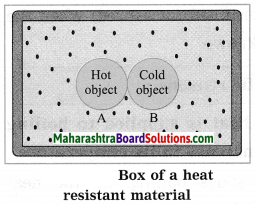Let m1 = mass of A, m2 = mass of B, c1 = specific heat capacity of A, c2 = specific heat capacity of B and T = common temperature attained by A and B after the heat exchange between A and B. Here, no heat leaves the box or enters the box from outside. Hence, if T1 > T2, heat energy lost by A (Q1) = heat energy gained by B (Q2).
∴ m1c1 (T1 – T) = m2c2 (T – T2)
[Note: If m1, c1, T1, T, m2 and T2 are known, c2 can be determined.]Question 33.
The specific heat capacity of silver is 0.056 kcal/kg·°C. Explain this statement.
The amount of heat needed to raise the temperature of 1 kg of silver by 1 °C is 0.056 kcal.

Question 34.
Explain how the specific heat capacity of a solid can be determined (measured) by the method of mixture.
A hot solid is put in water in a calorimeter. The mixture is stirred continuously and the maximum temperature of the mixture is measured with a thermometer. Heat exchange between the hot solid, water and calorimeter results in sill bodies attaining the same temperature after some time. Hence, according to the principle of heat exchange, heat lost by the solid = heat gained by the water in the calorimeter + heat gained by the calorimeter.

Now, heat lost by the solid (Q) = mass of the solid × its specific heat capacity × decrease in its temperature, heat gained by the water (Q1) = mass of the water × its specific heat capacity × increase in its temperature and heat gained by the calorimeter (Q2) = mass of the calorimeter × its specific heat capacity × increase in its temperature.

Heat lost by the hot object = heat gained by the calorimeter + heat gained by the water. Q = Q2 + Q1
Using this equation, the specific heat capacity of the solid can be determined (measured) when the other quantities are known.

Give scientific reasons:

Question 1.
Even though heat is supplied to boiling water, there is no increase in its temperature.
Once water starts boiling, all the heat supplied to it is used in conversion of water into steam at the boiling point of water. Hence, there is no rise in its temperature.

Question 2.
Burns from steam are worse those from boiling water at the same temperature.
1. A given quantity of steam contains more heat than the same quantity of boiling water at the same temperature.
2. When steam comes in contact with one’s body, it releases extra heat of 540 calories per gram and causes a more serious burn than that caused by boiling water.Question 3.
In winter, the pipelines carrying water burst in cold countries.
1. In cold countries, in winter, the temperature of the atmosphere falls below 0 °C. When the temperature of water falls below 4 °C, it expands. Hence, the water in pipes expands. Even if ice is formed, there is an increase in the volume.

2. As there is no room for expansion, water (or ice) exerts a large pressure on the pipes. Hence, the pipelines carrying water burst.

Question 4.
If crushed ice is pressed and then the pressure is released, a lump of ice is formed.
1. When crushed ice is pressed, its melting point is lowered and some ice melts to form water.
2. When pressure is released, the melting point becomes normal and the water freezes to form ice forming a lump.

Question 5.
In cold countries, in winter, even when the water of lakes freezes, aquatic animals and plants can survive.
1. In cold countries, in winter, a layer of ice is formed on the surface of lakes when the atmospheric temperature falls below 0 °C. However, below this layer, there is water at 4 °C.
2. Ice, being a bad conductor of heat, does not allow transfer of heat from this water to the atmosphere. Hence, aquatic animals and plants can survive in this water.

Question 6.
Water droplets are seen on’ the outer surface of a cold drink bottle.
1. The temperature of the outer surface of a cold drink bottle is less than that of the atmosphere.
2. Therefore, the excess of water vapour from the air condenses to form droplets on the outer surface of the cold drink bottle.

Question 7.
During cold nights, sometimes dew is formed.
1. During a cold night, the temperature of air may fall to the dew point, or even below the dew point. 2. If the temperature falls below the dew point, the excess of water vapour in air condenses on the surfaces of cold bodies and dew is formed.Question 8.
When you enter a warm room after being outside on a frosty early morning, your spectacles ‘steam up’.
1. On a frosty early morning, the temperature of air outside a warm room is lower than the dew point.
2. Hence, when you enter the room from outside, some water vapour in the room condenses on the glass of your spectacles, i.e., the spectacles ‘steam up’.

Question 9.
A plastic bottle, completely filled with water, when kept in a freezer, is likely to break.
The temperature of air in the freezer (deep freeze) compartment of a refrigerator is less than 0 °C. 2. When a plastic bottle, completely filled with water, is kept in this compartment, the temperature of water falls below 4 °C and the water expands. Even when water freezes and ice is formed, there is an increase in the volume. It exerts a large pressure on the sides of the bottle and hence the bottle is likely to break.

Question 10.
The outer surface of a beaker containing ice cubes becomes wet in a short while.
1. When ice cubes are placed in a beaker, ice starts melting. The heat required for melting is absorbed from the surrounding air and also from the beaker to some extent.
Hence, the temperature of the air and beaker falls.

2. The capacity of air to hold water vapour depends upon the temperature of the air, and this capacity decreases as the temperature decreases. At a certain low temperature, the surrounding air becomes saturated with water vapour present in it. As the temperature falls further, the air is unable to hold all the water vapour.

Hence, the extra water vapour starts condensing on the cold outer surface of the beaker in the form of minute drops. Therefore, the outer surface of the beaker containing ice cubes becomes wet in a short while.

Distinguish between the following:

Question 1.
Absolute humidity and Relative humidity.
Absolute humidity:

1. Absolute humidity is the mass of water vapour present in a unit volume of air.
2. It is commonly expressed in kg/m3.

Relative humidity:

1. Relative humidity is the ratio of the mass of water vapour in a given volume of air at a given temperature to the mass of water vapour required to saturate the same volume of air at the same temperature.
2. It does not have unit.

Solve the following examples/Numerical problems:
[Use the data given in the Tables on pages 130 and 131.]

Problem 1.
Calculate the amount of heat required to convert 5 g of ice of 0 °C into water at 0 °C. (Specific latent heat of fusion of ice = 80 cal/g)
Solution: Here, m = 5 g, L = 80 cal/g; Q = ?
Amount of heat required, Q = mL
= 5 g × 80 cal/g
= 400 calories.

Problem 2.
Find the amount of heat required to convert 10 g of water at 100 °C into steam. (Specific latent heat of vaporization of water = 540 cal/g)
Solution: Here, m = 10 g, L = 540 cal/g; Q = ?
Amount of heat required, Q = mL
= 10 g × 540 cal/g
= 5400 calories.Problem 3.
Calculate the amount of heat required to convert 15 g of water at 100 °C into steam. (Specific latent heat of vaporization of water = 540 cal/g)
Solution:
m = 15 g, L = 540 cal/g; Q = ?
Amount of heat required, Q = mL
= 15 g × 540 cal/g
= 8100 calories.

Problem 4.
How many calories of heat will be absorbed when 3 kg of ice at 0 °C melts?
Solution:
m = 3 kg = 3000 g; L = 80 cal/g; Q = ?
Quantity of heat absorbed, Q = mL
= 3000 g × 80 cal/g
∴ Q = 240000 calories.

Problem 5.
Calculate the amount of heat required to convert 10 g of water at 30 °C into steam at 100 °C. (Specific latent heat of vaporization of water = 540 cal/g)
Solution:
Here, m = 10 g; c = 1 cal/g·°C
T2 – T1 = 100 °C – 30 °C = 70 °C; L = 540 cal/g; Q = ?
Amount of heat required, Q = mc (T2 – T1) + mL
= 10 g × 1 cal/g·°C × 70 °C + 10 g × 540 cal/g
= 700 cal + 5400 cal
∴ Q = 6100 calories.

Problem 6.
If water of mass 80 g and temperature 45 °C is mixed with water of mass 20 g and temperature 30 °C, what will be the maximum temperature of the mixture?
Solution:
Data : m1 = 80 g, T1 = 45 °C, m2 = 20 g,
T2 = 30 °C, T = ?
According to the principle of heat exchange, heat lost by hot water = heat gained by cold water
∴ m1c (T1 – T) = m2c (T – T2)
∴ m1T1 – m1T = m2T – m2T2
∴ m1T1 + m2T2 = (m1 + m2)T
∴ Maximum temperature of the mixture,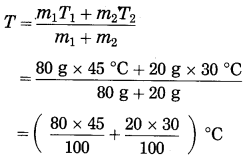= (36 + 6) °C
= 42°C.

Problem 7.
When water of mass 70 g and temperature 50 °C is added to water of mass 30 g, the maximum temperature of the mixture is found to be 41 °C. Find the temperature of water of mass 30 g before hot water was added to it.
Solution:
Data : m1 = 70 g, T1 = 50 °C, m2 = 30 g, T = 41 °C, T2 = ?
According to the principle of heat exchange, heat lost by hot water = heat gained by cold water
∴ m1c (T1 – T) = m2c (T – T2)
∴ m1T1 – m1T = m2T – m2T2
∴ m2T2 = (m1 + m2) T – m1T1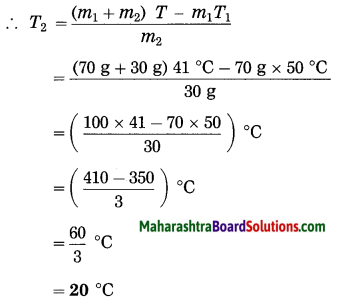This is the required temperature.Problem 8.
Find the heat needed to raise the temperature of a silver container of mass 100 g by 10 °C. (c = 0.056 cal/g.°C)
Solution:
Data: m = 100 g, ΔT = 10 °C, c = 0.056 cal/g·°C
Heat needed to raise the temperature of the container = mc ΔT
= 100 g × 0.056 cal/g·°C × 10 °C .
= 56 calories.

Problem 9.
If steam of mass 100 g and temperature 100 °C is released on an ice slab of temperature 0 °C, how much ice will melt?
Solution:
Data: m1 = 100 g, L1 = 540 cal/g,
T1 = 100 °C, mass of ice, m = ?, L2 = 80 cal/g, c (water) = 1 cal/g·°C
According to the principle of heat exchange, heat lost by hot body = heat gained by cold body. Conversion of steam into water:
Q1 = m1L1 = 100 g × 540 cal/g = 54000 cal
Decrease in the temperature of this water to 0 °C:
Q2 = m1c × (T1 – 0 °C) = 100 g × 1 cal/g·°C × (100 °C – 0 °C) = 10000 Cal
Melting of ice: Q3 = mL2
= m × 80 cal/g
Now, Q1 + Q2 = Q3
∴ (54000 + 10000) cal = m × 80 cal/g
∴ m = $$\frac{64000}{80}$$ = 800 g
800 g of ice will melt.

Numerical problems for practice:

Problem 1.
Calculate the amount of heat required to convert 80 g of ice at 0 °C into water at the same temperature. (Specific latent heat of fusion of ice = 80 cal/g)
Solution:
6400 calProblem 2.
Find the heat required to convert 20 g of ice at 0 °C into water at the same temperature. (Specific latent heat of fusion of ice = 80 cal/g)
Solution:
1600 cal

Problem 3.
Calculate the quantity of heat released during the conversion of 10 g of ice cold water (temperature 0 °C) into ice at the same temperature. (Specific latent heat of freezing of water = 80 cal/g)
Solution:
800 cal

Problem 4.
How many calories of heat will be absorbed when 2 kg of ice at 0 °C melts? (Specific latent heat of fusion of ice = 80 cal/g)
Solution:
160000 cal

Problem 5.
How much heat will be required to convert 20 g of water at 100 °C into steam at 100 °C? (Specific latent heat of vaporization of water = 540 cal/g)
Solution:
10800 cal

Problem 6.
Find the heat absorbed by 25 g of water at 100 °C to convert into steam at the same temperature. (Specific latent heat of vaporization of water = 540 cal/g.)
Solution:
13500 cal

Problem 7.
If water of mass 60 g and temperature 50 °C is mixed with water of mass 40 g and temperature 30 °C, what will be the maximum temperature of the mixture?
Solution:
42 °C

Problem 8.
If water of mass 60 g and temperature 60 °C is mixed with water of mass 60 g and temperature 40 °C, what will be the maximum temperature of the mixture?
Solution:
50 °C

Problem 9.
Find the heat needed to raise the temperature of a piece of iron of mass 500 g by 20 °C. (c = 0.110 cal/g·°C)
Solution:
1100 cal

Problem 10.
Water of mass 200 g and temperature 30 °C is taken in a copper calorimeter of mass 50 g and temperature 30 °C. A copper sphere of mass 100 g and temperature 100 °C is released into it. What will be the maximum temperature of the mixture? [c (water) = 1 cal/g·°C, c (copper) =0.1 cal/g·°C]
Solution:
33.26 °CProblem 11.
A copper calorimeter of mass 100 g and temperature 30 °C contains water of mass 200 g and temperature 30 °C. If a piece of ice of mass 40 g and temperature 0 °C is added to it, what will be the maximum temperature of the mixture? [c (copper) = 0.1 cal/g·°C, c (water) = 1 cal/g·°C, L = 80 cal/g]
Solution:
12.4 °C